## A powerful graphics library for great visualizations

Do you wish that Python could emulate the superb visualizations that ggplot gives you in the R language? Well it can.

We are going to explore the capabilities of Plotnine, a visualization library for Python that is based on ggplot2.

Being able to visualize your data gives you the ability to better understand it. It gives you the opportunity to gain insights into the relationships between elements of that data and to spot correlations and dependencies. ggplot, in R, and Plotnine in Python gives you the ability to do this in a logical way.

You don't need to be an expert in Python to be able to do this, although some exposure to programming in Python would be useful, as would be a basic understanding of DataFrames in Pandas.

It would be helpful if you are familiar with Jupyter Notebooks, too.

### ggplot

ggplot2 is a powerful graphics library for R and is described in the book "ggplot2: Elegant Graphics for Data Analysis" by Hadley Wickham. Wickham, in turn, based his work on "The Grammar of Graphics", a book by Leland Wilkinson. Wilkinson’s book gives the theoretical basis for a grammar of graphics and Wickham’s book shows how such a grammar can be implemented (in ggplot2).

ggplot2 implements a layered approach to constructing graphics and allows the possibility of either using standard routines for the construction of popular graphs and charts, or the construction of custom graphics to suit your own purposes.

Plotnine is based on ggplot2 and implemented in Python.

Depending on your Python installation, you can install it with pip:

pip install plotnine

or Conda:

conda install -c conda-forge plotnine

This article will focus on how to construct and customize standard graphs - lines, bars and so on - use layers to modify those plots and hopefully give an inkling as to how you could produce customized plots of your own.

from plotnine import *

### Layers

There are three basic elements to a ggplot command, data, aesthetics and layers. The role of data is clear and we should provide it in the form of a Pandas DataFrame. Aesthetics are where variables in the data are mapped onto visual properties and layers describe how to render the data, for example, as a bar chart. There can be several layers that define different charts or parts of the chart, such as labels on the axes.

In R commands in ggplot2 look something like:

ggplot(data, aesthetics)
+ layer1()
+ layer2()

Plotnine uses the same pattern but this doesn't fit with Python syntax very well. You could write:

ggplot(data,aesthetics) + layer1() + layer2()

but you can end up with very long lines. The solution is simple, though. Just enclose the whole thing in braces. So we end up with:

(ggplot(data,aesthetics)
+ layer1()
+ layer2()
)

So this is the style that I will use in this article.

### Getting Data

If you've followed my introductions to visualization for Pandas Plot or Julia, you will be familiar with the weather data that I use. It is public data derived from the UK Meteorological Office and charts weather data for London over the last few decades.

The data tracks the maximum and minimum temperatures, the number of hours of sunshine and the rainfall for each month. There are two tables, one is the complete set of data from 1957 and a shorter one records the data for 2018, only.

We’ll get the 2018 data first.

import pandas as pd

weather

And this it what it looks like: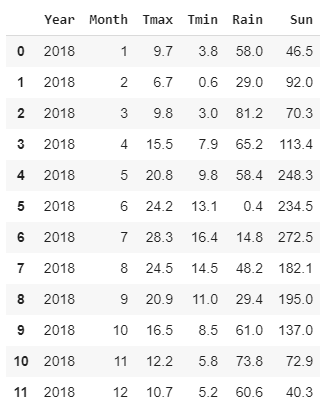The Year column is fairly redundant but is consistent with the larger data file. The other columns are self-explanatory. Months are numbered from 1 to 12, temperatures are in Celsius, rainfall is millimeters and sunshine is in hours.

So, to get started with ggplot, we are going to draw a line graph of the maximum temperatures for each month. We will then add some layers to enhance the graph.

### Line graph

The anatomy of the call to ggplot is as described above. The first parameter is the data that we are going to graph, weather, the next parameter is a call to aes. aes maps the data onto various ‘aesthetics’ - here we have just two. By default, the first two parameters are the x and y axes. So here Month will be on the x axis and Tmax on the y axis.

This by itself is a legitimate call to ggplot but it won’t draw anything. To do that we need to add a layer to tell ggplot what sort of graph we want. Graph types are called geoms and the one we use here is geom_line, which is, of course a line graph.

(ggplot(weather,aes('Month', 'Tmax'))
+ geom_line()
)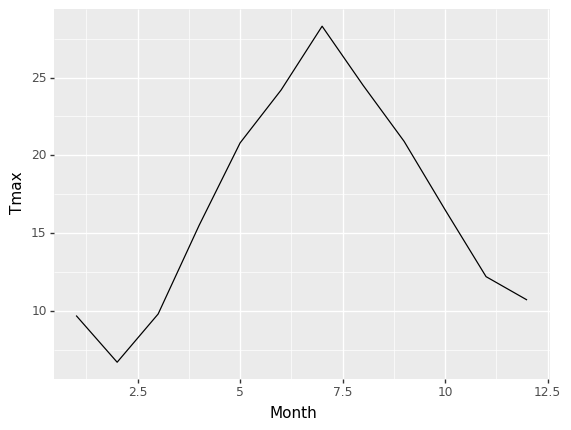That's a good start but we can do better with a couple of modifications. For example, you'll notice that the months are numeric values rather than actual dates, so the line plot has interpreted them as real numbers. This means that we end up with the months ranging from 2.5 to 12.5 - not ideal.

We can easily fix this by adding another layer to the graph function. ggplot allows us to specify the ticks each axis, so we'll add a layer to do that. We want the ticks to be the numbers 1 through 12, which is exactly what is in the Month column. So we'll use that data to tell ggplot what the ticks should be.

For convenience I am going to use a variable months. Like this:

months = weather['Months']

Here is the complete code

months=weather['Month']

(ggplot(weather,aes('Month','Tmax'))
+ geom_line()
+ scale_x_continuous(breaks=months)
)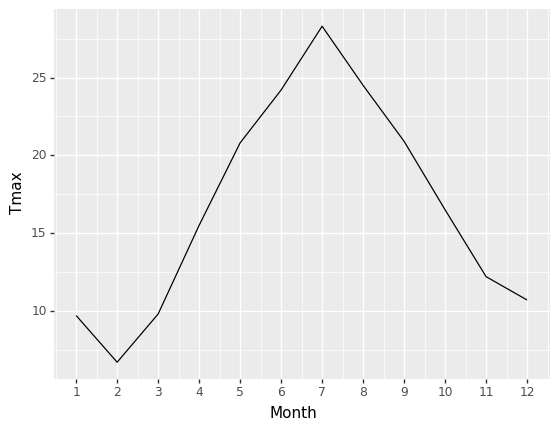Also, I’m not keen on the default color scheme. ggplot comes with a number of themes that we can use, so I’ll add another layer to specify that I want to use the Light theme and add a parameter to geom_line to tell it to draw a red line instead of the default black one.

month_labels=("Jan","Feb","Mar","Apr","May","Jun","Jul","Aug","Sep","Oct","Nov","Dec")

(ggplot(weather,aes('Month','Tmax'))
+ geom_line(color='red')
+ scale_x_continuous(breaks=months,labels=month_labels)
+ theme_light())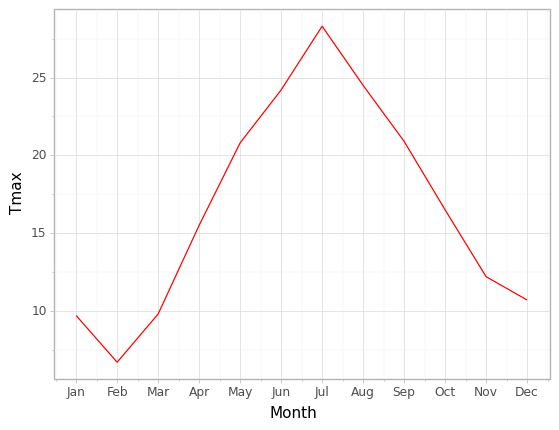That’s an improvement, I think.

Let’s do the same sort of plot but with a column chart.

### Column chart

To draw a column chart we simply swap the geom. Instead of geom_line, we use geom_col. Simple. The only thing to watch out for is that if we want to change the color of the bars, we need to specify a ‘fill’. There is a parameter ‘color’ but this only changes the outline of the columns.

(ggplot(weather,aes('Month','Tmax'))

+ geom_col(fill='red')

+ scale_x_continuous(breaks=months, labels=month_labels)

+ theme_light()

)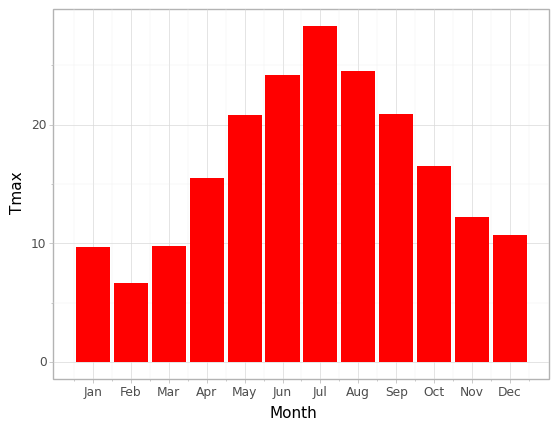And here is the same sort of thing for Rain - with a suitably rainy color change.

(ggplot(weather,aes('Month','Rain'))
+ geom_col(fill='green')
+ scale_x_continuous(breaks=months, labels=month_labels)
+ theme_light()
)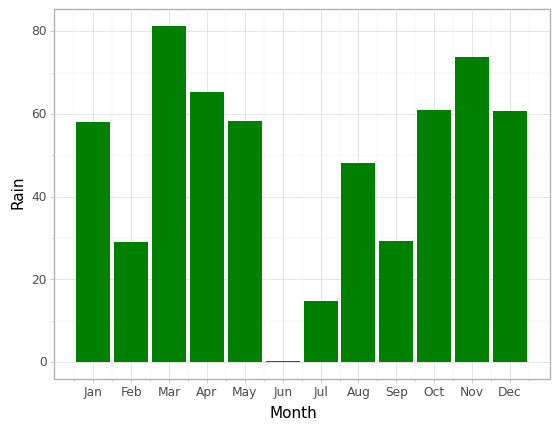### Multiple graphs

I want to draw Tmax and Tmin on the same graph - how do I do that?

The best way is to transform the data so that the two temperatures are in the same column and labelled as either Tmax or Tmin in a separate column. This is easily done with the Pandas melt function. Here I have created a new dataframe temps with the required columns.

temps = pd.melt(weather, id_vars=['Month'], value_vars=['Tmax','Tmin'],

var_name='Temp', value_name='DegC' )
temps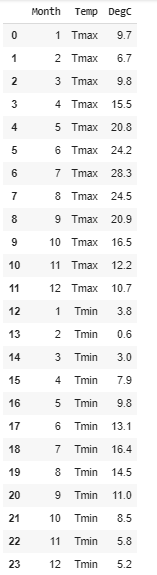And now I plot them with a column geom. There are a couple of things to note here. First, I’ve taken the fill out of the geom and put it in the call to aes and assigned it to Temps (which will either be Tmax or Tmin).

Specifying a color in the geom fixes that color in that geom, whereas by putting it in aes I can tell the geom to color the columns differently for each Temp value. So the column geom will now color two separate bars one for Tmax and the other for Tmin. By default these bars would be stacked but here we want them to be side by side.

So the second thing is that in the column geom I’ve specified the position to be ‘dodge’ which gives us the side by side configuration.

(ggplot(temps,aes('Month','DegC',fill='Temp'))
+ geom_col(position='dodge')
+ scale_x_continuous(breaks=months)
+ theme_light()
)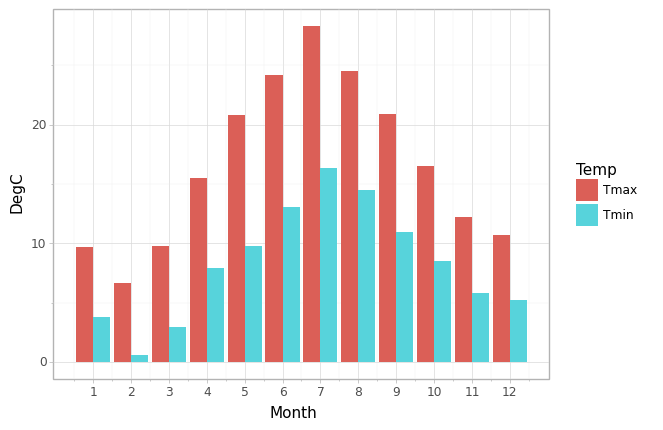Here is the same thing but with lines. Note that I specify color not fill for the lines and that I don’t need to worry about the position.

(ggplot(temps,aes('Month','DegC',color='Temp'))
+ geom_line()
+ scale_x_continuous(breaks=months)
+ theme_light()
)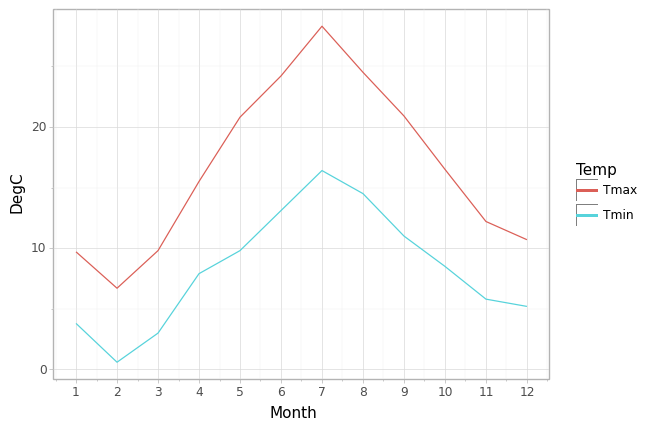### More layers - labels

As you have seen, to add more to the plot, we add more layers. To modify the labels on our chart we can do the same. In the next piece of code I’ve added layers to specify the captions on the x and y axes, and have given the whole chart a title.

(ggplot(temps,aes('Month','DegC',color='Temp'))
+ geom_line()
+ scale_x_continuous(breaks=months)
+ theme_light()
+ xlab('2018')
+ ylab('Temperature in degrees C')
+ ggtitle('Monthly Maximum and Minimum Temperatures')
)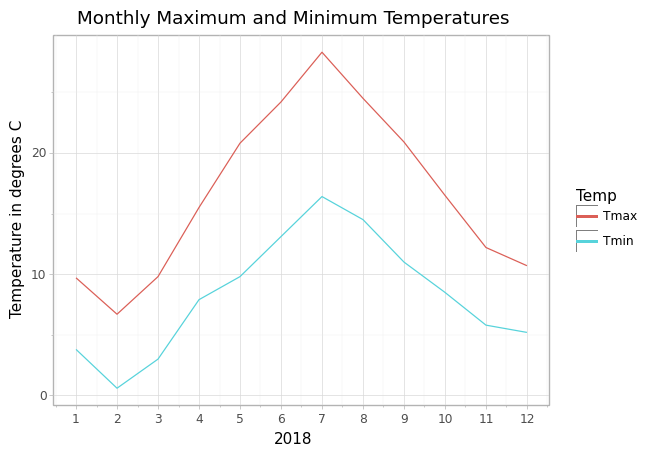### More layers - Facets

Let’s think about drawing a single figure that summarizes the entire table of data. Column charts for the two temperatures, for rainfall and sunshine.

First, we melt the dataframe again but this time we put all of the data in a single column. Each value will be labelled as Tmax, Tmin, Rain or Sun.

data = pd.melt(weather, id_vars=['Month'], value_vars=['Tmax','Tmin','Rain','Sun'], var_name='Measure', value_name='Value' )

To create the faceted graph we add a facet_wrap layer and we pass the Measure to it meaning that we will get facets for each of Tmax, tmin, Rain and Sun. By default, the facets will all use the same scale, which would be fine for Tmax and Tmin. But Rain and Sun are both different from each and the temperatures. So we need to tell facet_wrap that the scales are ‘free’, that is, each facet will have its own scale.

The trouble is that doing this means that the labels for the y axes tend to overlap the charts, so we need to adjust the layout with another layer. The last layer is a modification of the theme (theme_light) to add extra spacing between the facets and to set the overall size of the figure. Note that this must come after the theme_light layer, otherwise theme_light will reset the layout to it default settings.

(ggplot(data, aes('Month','Value', fill='Measure'))
+ geom_col(show_legend=False)
+ scale_x_continuous(breaks=months,labels=month_labels)
+ facet_wrap('Measure', scales='free')
+ xlab('')
+ ylab('')
+ ggtitle('Weather Data for London, 2018')
+ theme_light()
+ theme(panel_spacing=0.5, figure_size=(10,5))
)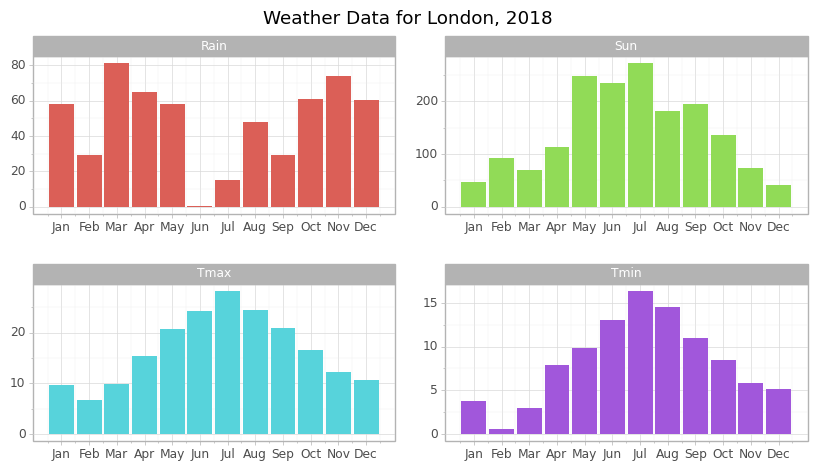(ggplot(weather2,aes('Sun','Tmax',color='Month'))
+ geom_point()
+ theme_light()
)

### Conclusion

This has been a fairly random walkthrough of some of the aspects and features of ggplot as implemented in Python by Plotnine. I hope that you can see the power of this approach and encourage you to read Hadley Wickham’s book on ggplot2 and see the Plotnine documentation